# ILP Part 40 — Ternary logic

This is the fortieth part of the ILP series. For your convenience you can find other parts in the table of contents in Part 1 – Boolean algebra

We already know how to implement binary logic in ILP, today we are going to utilize it to implement ternary logic.

# Implementation

Zero and one (or false and true) are rather ordinary, third value is called null or unknown and represent situation when we don’t know exact value. It usually has value. Truth tables for ternary logic go as follows:
Disjunction: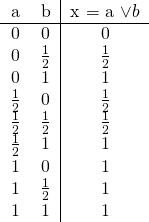Conjunction: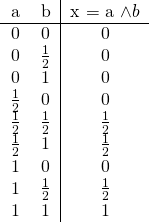We are going to represent any ternary logic value as a pair of boolean flags. You can intuitively treat this as a number in binary system with values multiplied by two, so false is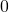, unknown is, and true is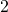. We know that disjunction can be calculated as a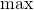function and conjunction as afunction and in theory we can implement operations in this way. However, simply checking all cases is faster. This is disjunction: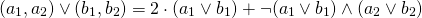Conjunction goes this way: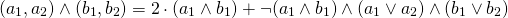Of course we need to destructure result to have two boolean flags at the end.